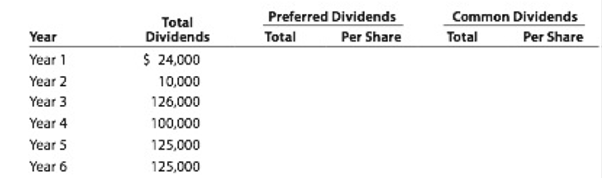Chapter 13, Problem 13.1BPR

Chapter
Section
Textbook Problem

Dividends on preferred and common stockYosemite Bike Corp. manufactures mountain bikes and distributes them through retail outlets in California, Oregon, and Washington. Yosemite Bike Corp. has declared the following annual dividends over a six-year period ended December 31 of each year: Year 1, $24,000; Year 2,$10,000; Year 3, $126,000; Year 4,$100,000; Year 5, $125,000; and Year 6, 5125,000. During the entire period, the outstanding stock of the company was composed of 25,000 shares of cumulative preferred 2% stock,$90 par, and 100,000 shares of common stock, $4 par.Instructions 1. Determine the total dividends and the per-share dividends declared on each class of stock for each of the six years. There were no dividends in arrears on January 1, Year 1. Summarize the data in tabular form, using the following column headings: 1.2. Determine the average annual dividend per share for each class of stock for the six- year period. 3. Assuming a market price of$100 for the preferred stock and $5 for the common stock, calculate the average annual percentage return on initial shareholders' investment, based on the average annual dividend per share (a) for preferred stock and (b) for common stock. 1. To determine Cash dividends: The amount of cash provided by a corporation out of its distributable profits to its shareholders as a return for the amount invested by them is referred as cash dividends. Common stock: These are the ordinary shares that a corporation issues to the investors in order to raise funds. In return, the investors receive a share of profit from the profits earned by the corporation. The dividend payments are not guaranteed and are paid after the payment made to the preferred stockholders. Preferred stock: The stock that provides a fixed amount of return (dividend) to its stockholder before paying dividends to common stockholders is referred as preferred stock. A preferred stock may be cumulative and non-cumulative. A cumulative preferred stock implies that a preferred stockholder is entitled to receive dividends for the current year plus any unpaid dividends of the previous years, before the dividends paid to the common stockholders. Dividend per share: Dividend per share represents the amount of dividend paid to each shareholders of the business. To determine: The total dividends and the amount of dividends declared per share for preferred and common stock for each of the six years. Explanation Determine the total dividends and the amount of dividends declared per share for preferred and common stock for each of the six years.  Year Total dividends Preferred dividends Common dividends Total Per share Total Per share 1$ 24,000 $24,000$ 0.96 $0$ 0 2 $10,000$ 10,000 $0.40$ 0 $0 3$ 126,000 $101,000$ 4.04 $25,000$ 0.25 4 $100,000$ 45,000 $1.80$ 55,000 $0.55 5$ 125,000 $45,000$ 1.80 $80,000$ 0.80 6 $125,000$ 45,000 $1.80$ 80,000 \$ 0.80

Table (1)

Working note:

Calculate the amount to be paid as preferred dividend each year...

2.

To determine
The average annual dividend per share for each class of stock for the six-year period.

3.

To determine
The average annual percentage return on initial stockholders’ investment, based on the average annual dividend per share for (A) preferred stock and (B) common stock.

Still sussing out bartleby?

Check out a sample textbook solution.

See a sample solution

The Solution to Your Study Problems

Bartleby provides explanations to thousands of textbook problems written by our experts, many with advanced degrees!

Get Started

Explain the four types of corporate cultures.

Foundations of Business (MindTap Course List)

What is inflation and what causes it?

Essentials of Economics (MindTap Course List)

AFN EQUATION Refer to Problem 16-1 and assume that the company had 3 million in assets at the end of 2014. Howe...

Fundamentals of Financial Management, Concise Edition (with Thomson ONE - Business School Edition, 1 term (6 months) Printed Access Card) (MindTap Course List)